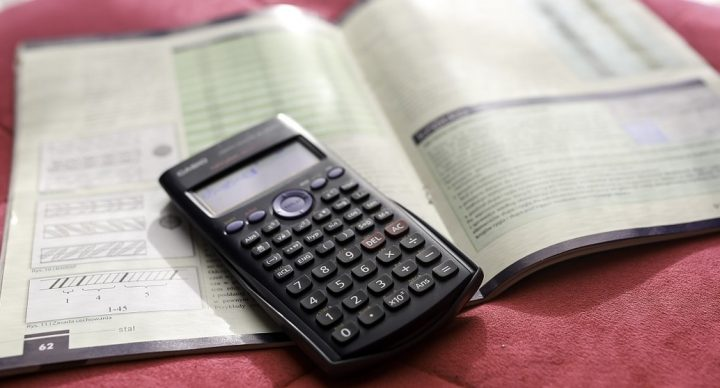# College Exam Math Prep### Course Overview

The Acellus College Exam Math Preparation course has been specially developed to help students prepare to take the math portion of the ACT or SAT college entrance exam. Students watch as problems similar to those presented in these exams are solved in the high-quality video lessons. After each lesson students are presented with several similar problems to enable them to practice the concepts that they have just reviewed. It is taught by award-winning Acellus Master Teacher, Patrick Mara. Course topics include:
• Sets
• Number Bases
• Fractions, Decimals, and Percentages
• Permutations and Combinations
• Linear Equations
• Polynomials
• Application Problems
• Exponents
• Distance and Mid-Point Formulas
• Conic Sections
• Systems of Equations
• Exponential and Logarithmic Functions
• Sequences and Series
• Complex Numbers
• Polygons
• Pythagorean Theorem
• Special Right Triangles (30-60-90 and 45-45-90)
• Area and Perimeter
• Trigonometric Functions
• Graphs of Trigonometric Functions
This course is an excellent review for all students preparing to take either the ACT or SAT. Students completing the Acellus College Exam Math Preparation course should be well prepared to take the math portion of these very important exams.

### Course Objectives & Student Learning OutcomesThis course was developed by the International Academy of Science. Learn More